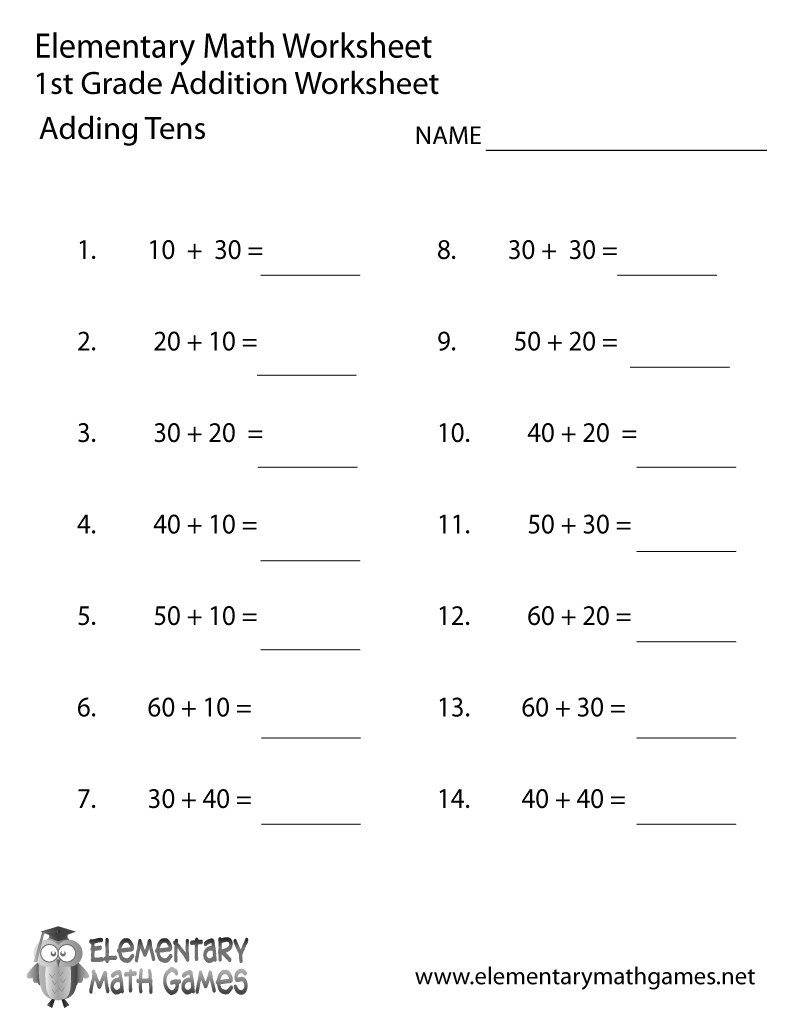## Learn and Practice How to Add Tens

This first grade math worksheet is a great way for kids to learn addition. There are 14 math problems for first graders to solve.

Here are the two versions of this free first grade math worksheet: